# 5G NR Downlink CSI Reporting

The example shows how to compute downlink channel state information (CSI) parameters such as the channel quality indicator (CQI), precoding matrix indicator (PMI) for multiple input multiple output (MIMO) scenarios, and rank indicator (RI), as defined in TS 38.214 Section 5.2.2, over a tapped delay line (TDL) channel. The example supports computation of the CSI parameters considering type 1 single panel or type 1 multipanel codebooks.

### Introduction

CSI parameters are the quantities related to the state of a channel. The user equipment (UE) reports CSI parameters to the access network node (gNB) as a feedback. The CSI feedback includes several parameters, such as the CQI, the PMI with different codebook sets, and the rank indicator (RI). The UE uses channel state information reference signal (CSI-RS) to measure the CSI feedback. Upon receiving the CSI parameters, the gNB schedules downlink data transmissions (such as modulation scheme, code rate, number of transmission layers, and MIMO precoding) accordingly. The figure shows an overview of CSI-RS transmission, CSI computation and feedback, and the transmission of downlink data that is scheduled based on the CSI parameters.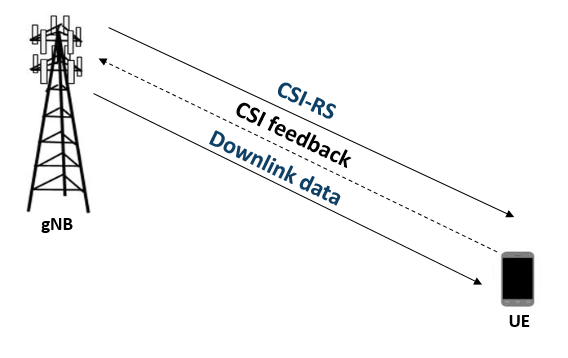#### RI Selection

The RI defines the number of possible transmission layers for the downlink transmission under specific channel conditions. It is also termed as the maximum number of uncorrelated paths that can be used for the downlink transmission. Other CSI parameters like the PMI and CQI are computed based on the rank provided by the RI. For given channel conditions, `hRISelect` computes the signal to interference and noise ratio (SINR) along with the PMI for all possible valid number of transmission layers. The function reports the number of transmission layers with the maximum total SINR as rank.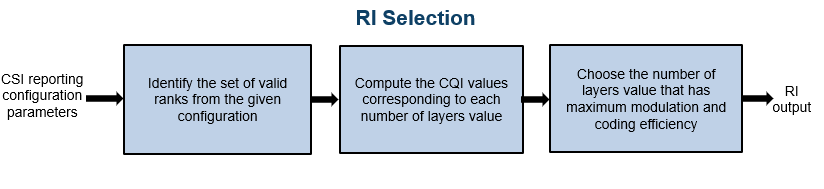#### PMI Selection

The PMI is a set of indices corresponding to a precoding matrix and gNB can apply this precoding matrix for the downlink data transmission. The `hDLPMISelect` selects a codebook from a set of possible codebooks, defined in TS 38.214 Tables 5.2.2.2.1-1 to 5.2.2.2.1-12 for type 1 single panel or TS 38.214 Tables 5.2.2.2.2-1 to 5.2.2.2.2-6 for type 1 multipanel, based on the codebook type, possible number of transmission layers for the downlink transmission and CSI reporting configuration parameters such as antenna panel dimensions and the codebook mode. Each codebook consists of a set of precoding matrices. For given channel conditions, the function computes SINR at the receiver side by considering all precoding matrices from the selected codebook. The function reports PMI as the set of indices (constituting the two indices set ${\mathit{i}}_{1},{\mathit{i}}_{2}\text{\hspace{0.17em}},$ as defined in TS 38.214 Section 5.2.2.2.1 for type 1 single panel codebooks and TS 38.214 Section 5.2.2.2.2 for type 1 multipanel codebooks) corresponding to a precoding matrix, which gives the maximum SINR. The figure shows the procedure for PMI selection.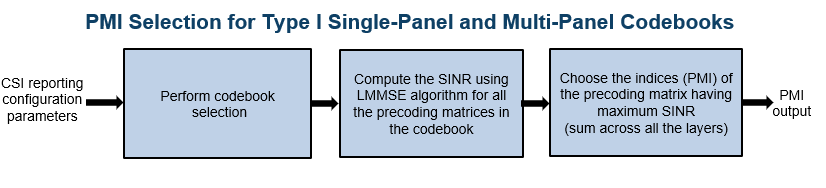#### CQI Selection

The CQI is an indicator of channel quality. The CQI index is a scalar value from 0 to 15. It provides the information about the highest modulation scheme and the code rate (MCS) suitable for the downlink transmission to achieve the required block error rate (BLER) for given channel conditions.

The CSI-reference resource is a group of downlink frequency-domain and time-domain resources that are configured, as defined in TS 38.214 Section 5.2.2.5. The gNB transmits a single physical downlink shared channel (PDSCH) transport block occupying the resource blocks termed as CSI reference resource, with a combination of modulation scheme and target code rate that correspond to each CQI index. The UE selects the highest index as CQI, when the mentioned PDSCH transport block can be received with a transport block error probability not exceeding:

• 0.1 for 'cqi-Table' as 'table1' or 'table2'

• 0.00001 for 'cqi-Table' as 'table3'

The 'cqi-Table' is a higher layer parameter that corresponds to the CQI versus MCS table, and SINR lookup table is computed for this table. This example uses a 0.1 BLER (corresponding to a 90 percent throughput) and 'cqi-Table' as 'table1' (TS 38.214 Table 5.2.2.1-2). The relationship between the CQI indices, the modulation scheme, and the code rate (from which the transport block size is derived) is described in TS 38.214 Tables 5.2.2.1-2 to 5.2.2.1-4.

The `hCQISelect` function computes the CQI value by considering the SINR values that correspond to the reported PMI, using the precalculated lookup table of the CQI index versus ${\mathrm{SINR}}_{\mathit{i}}$, as a reference. The function maps the SINR values across all layers (corresponding to the reported PMI) to each codeword. For each codeword, the function compares the respective SINR with the ${\mathrm{SINR}}_{\mathit{i}}$ values from the table and then, selects the CQI value against the maximum SINR, which is less than the codeword SINR. The CQI value is set such that the BLER is less than or equal to 0.1 when operated at ${\mathrm{SINR}}_{\mathit{i}}$. If a CQI index of 1 does not satisfy the BLER condition, then the CQI index is set as 0. The figure shows the procedure for CQI selection.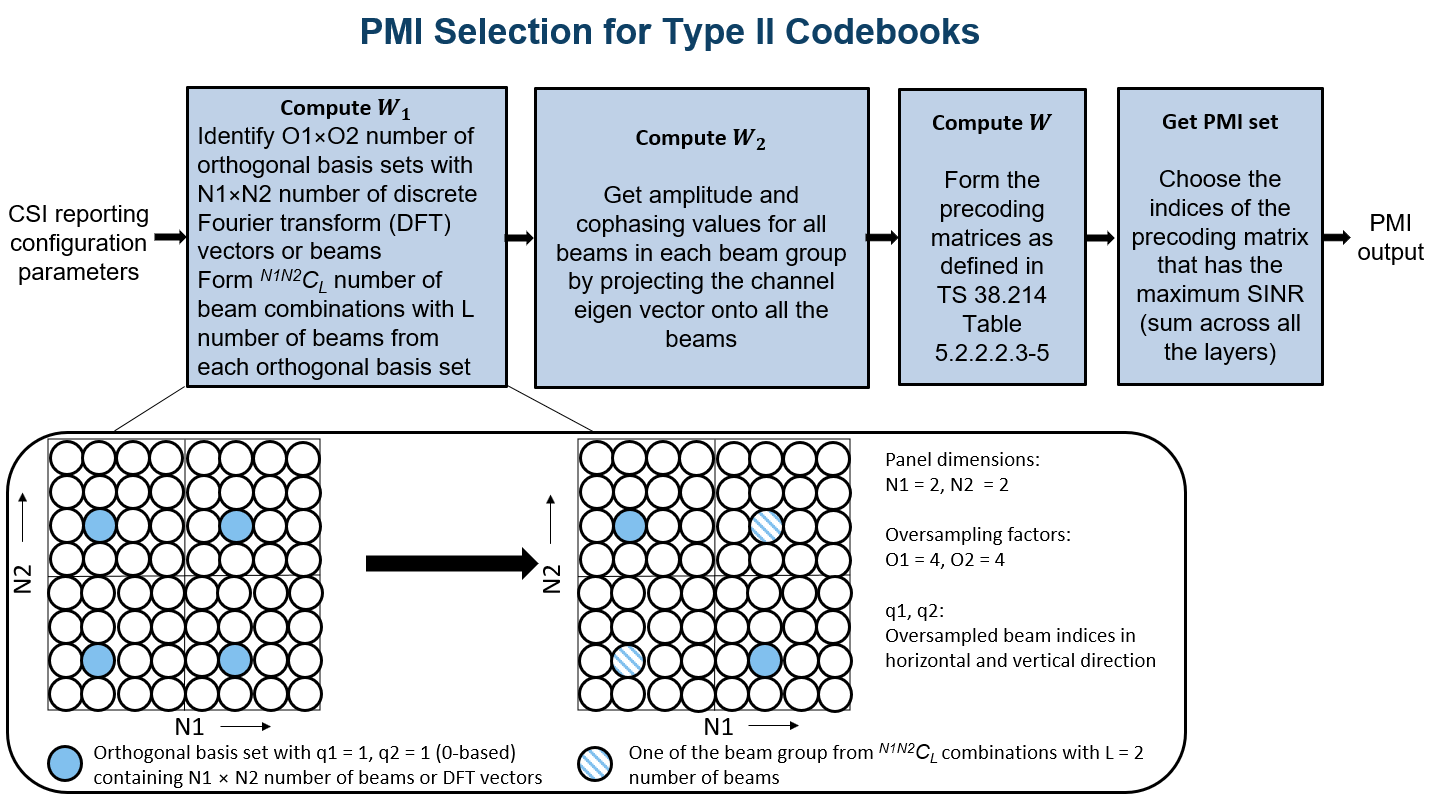#### CQI Versus SINR Table

To create the lookup tables, update the `NewRadioPDSCHThroughputExample` to configure the CSI reference resource, as defined in TS 38.214 Section 5.2.2.5. Perform simulations for PDSCH throughput by considering the modulation scheme and code rate corresponding to each CQI, certain channel conditions and across a finite range of SINR values with zero interference. Map the SINR value observed at the receiver, where the target BLER is achieved, against each CQI index. The figure shows the procedure for CQI versus SINR table creation.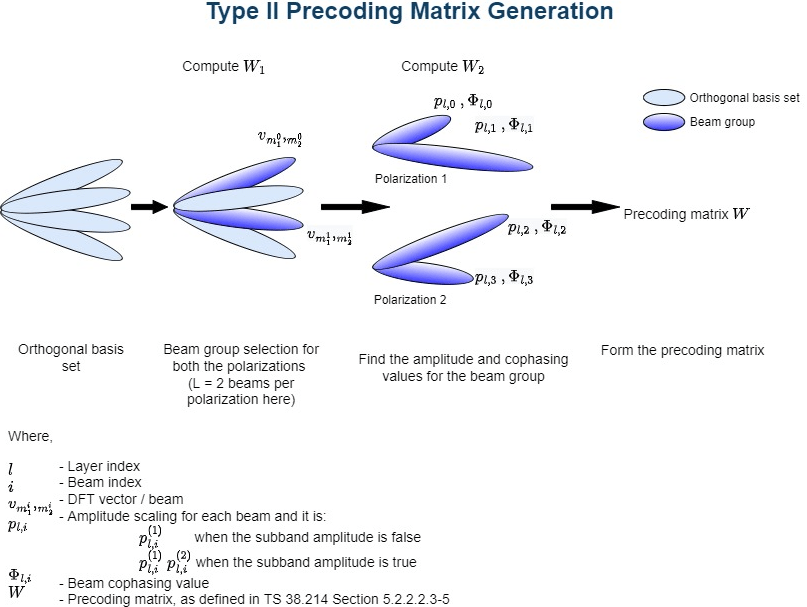#### Outline of Example

This example shows how to compute the CQI and PMI indices for a 4-by-4 MIMO scenario over a TDL channel with delay profile TDL-C, a delay spread of 300e-9 seconds, and a maximum Doppler shift of 50 Hz. It compares the time-domain and frequency-domain variations of CQI values against the time-domain and frequency-domain variations of SINR values for both practical and perfect channel estimation scenarios. The example also highlights the time-domain and frequency-domain variations of the PMI values for practical and perfect channel estimation scenarios corresponding to the reported rank.

### Simulation Length and SNR Point

Set the length of the simulation in terms of the number of 10 ms frames. The SNR (SINR with zero interference) is defined per resource element (RE) and applies to each receive antenna. For an explanation of the SNR definition that this example uses, see SNR Definition Used in Link Simulations.

```nFrames = 5; % Number of 10 ms frames SNRdB = 10; % SNR in dB```

### Carrier, Bandwidth Part, and CSI-RS Configuration

Create a carrier configuration object representing a 10 MHz carrier with a subcarrier spacing of 15 kHz.

```carrier = nrCarrierConfig; carrier.SubcarrierSpacing = 15; carrier.NSizeGrid = 52;```

Configure the size of the bandwidth part (BWP) and the start of the BWP relative to common resource block 0 (CRB 0).

```NStartBWP = 0; NSizeBWP = 52;```

Create a CSI-RS configuration object representing a non-zero-power CSI-RS (NZP-CSI-RS) resource set with a set of NZP-CSI-RS resources. Make sure that the NZP-CSI-RS resources that are configured in this example have the same code division multiplexing (CDM) types and the same number of CSI-RS ports, as defined in TS 38.214 Section 5.2.2.3.1.

```csirs = nrCSIRSConfig; csirs.CSIRSType = {'nzp','nzp','nzp'}; csirs.RowNumber = [4 4 4]; csirs.NumRB = 52; csirs.RBOffset = 0; csirs.CSIRSPeriod = [4 0]; csirs.SymbolLocations = {0, 0, 0}; csirs.SubcarrierLocations = {0, 4, 8}; csirs.Density = {'one','one','one'}; ```

#### Other Simulation-wide Parameters

Configure the number of transmit and receive antennas. The number of transmit antennas must be equal to the number of CSI-RS ports because the antenna ports to physical antenna mapping is not considered in this example.

```nTxAnts = csirs.NumCSIRSPorts(1); nRxAnts = 4;```

Validate the CSI-RS configuration object.

`validateCSIRSConfig(carrier,csirs,nTxAnts);`

### CSI Reporting Configuration

Specify the parameters required for the CQI, PMI, and RI computation as a structure with fields:

• `NSizeBWP`: Size of the BWP in terms of the number of physical resource blocks (PRBs)

• `NStartBWP`: Starting PRB index of the BWP relative to CRB 0

• `CodebookType`: The type of codebooks according to which the CSI parameters must be computed (`'Type1SinglePanel'`, `'Type1MultiPanel'`)

• `PanelDimensions`: The antenna panel dimensions corresponding to the `CodebookType` field.

If `CodebookType` is configured as `'Type1SinglePanel'`, the panel dimensions are configured as [N1 N2] according to TS 38.214 Table 5.2.2.2.1-2, as shown in the figure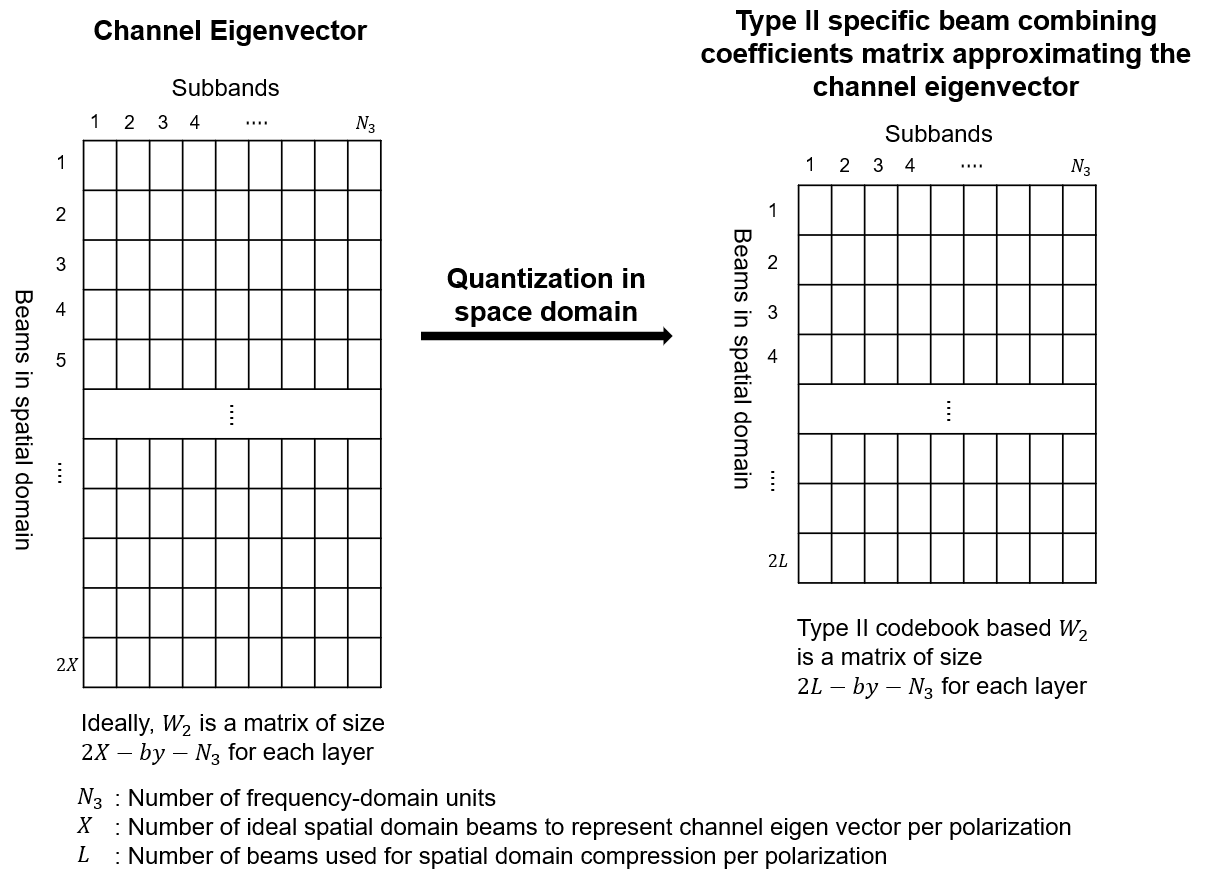If `CodebookType` is configured as '`Type1MultiPanel'`, the panel dimensions are configured as [Ng N1 N2] according to TS 38.214 Table 5.2.2.2.2-1, as shown in the figure• `CQIMode`: The mode of CQI reporting (`'Subband'`, `'Wideband'`)

• `PMIMode`: The mode of PMI reporting (`'Subband'`, `'Wideband'`)

• `SubbandSize`: The size of the subband, as defined in TS 38.214 Table 5.2.1.4-2 (required only when `CQIMode` or `PMIMode` is `'Subband'`)

• `PRGSize`: The precoding resource block group (PRG) size for the CQI calculation (required only when the reporting must be done for the report quantity 'cri-RI-i1-CQI' and `CodebookType` is configured as `'Type1SinglePanel'`, as defined in TS 38.214 Section 5.2.1.4.2)

• `CodebookMode`: The codebook mode according to which the codebooks are derived. When the `CodebookType` is configured as `'Type1SinglePanel'`, this field is required only when the number of transmission layers is 1 or 2 and the number of CSI-RS ports is greater than 2.

• `CodebookSubsetRestriction`: Restriction parameter pertaining to the codebook index set ${\mathit{i}}_{1}$, that is, ${\mathit{v}}_{\mathrm{lm}}$ or ${\stackrel{~}{\mathit{v}}}_{\mathrm{lm}}$ restriction parameter, as defined in TS 38.214 Section 5.2.2.2.1. This field denotes the set of ${\mathit{i}}_{1}$ indices ($\left[{\mathit{i}}_{11\text{\hspace{0.17em}}},\text{\hspace{0.17em}}{\mathit{i}}_{12\text{\hspace{0.17em}}\text{\hspace{0.17em}}}\right]$) that are restricted from the consideration for the PMI computation.

• `i2Restriction`: Restriction parameter pertaining to the codebook index ${\mathit{i}}_{2}$. This field denotes the set of ${\mathit{i}}_{2}$ indices that are restricted from the consideration for the PMI computation (applicable only when `CodebookType` is `'Type1SinglePanel'`).

• `RIRestriction`: Restriction parameter pertaining to the rank indicator, which implies the set of ranks that are restricted from usage for the RI computation.

```% Configure CSI reporting configuration parameters reportConfig.NStartBWP = NStartBWP; reportConfig.NSizeBWP = NSizeBWP; reportConfig.CodebookType = 'Type1SinglePanel'; reportConfig.PanelDimensions = [2 1]; reportConfig.CQIMode = 'Subband'; reportConfig.PMIMode = 'Subband'; reportConfig.SubbandSize = 4; reportConfig.PRGSize = []; reportConfig.CodebookMode = 1; reportConfig.CodebookSubsetRestriction = []; reportConfig.i2Restriction = []; reportConfig.RIRestriction = [];```

### Propagation Channel Model Configuration

Consider a TDL channel with delay profile TDL-C, a delay spread of 300e-9 seconds, and a maximum Doppler shift of 50 Hz.

```% Create channel object channel = nrTDLChannel; channel.NumTransmitAntennas = nTxAnts; channel.NumReceiveAntennas = nRxAnts; channel.DelayProfile = 'TDL-C'; channel.MaximumDopplerShift = 50; channel.DelaySpread = 300e-9;```

Set the sampling rate for the channel model by using the value returned from the `nrOFDMInfo` function.

```waveformInfo = nrOFDMInfo(carrier); channel.SampleRate = waveformInfo.SampleRate;```

Get the maximum number of delayed samples by a channel multipath component. The maximum number of delayed samples is calculated from the channel path with the largest delay and the implementation delay of the channel filter. The number of samples that correspond to the maximum channel delay is required later to flush the channel filter to obtain the received signal.

```chInfo = info(channel); maxChDelay = ceil(max(chInfo.PathDelays*channel.SampleRate)) + chInfo.ChannelFilterDelay;```

### Processing Loop

For each slot, generate the CSI-RS, transmit through the channel, and then process at the receiver side to compute the CQI, PMI, and RI values. Follow the steps for the transmit to receive end processing for each slot.

1. Generate the resource grid: Generate the slot grid that is populated with the CSI-RS and map the slot grid from CSI-RS ports to transmit antennas.

2. Generate the waveform: Perform orthogonal frequency division multiplexing (OFDM) modulation on the generated grid by using the `nrOFDMModulate` function.

3. Model and apply a noisy channel: Pass the waveform through a TDL-C fading channel, and then add the additive white Gaussian noise (AWGN). The SNR is defined per RE and applies to each receive antenna.

4. Perform timing synchronization and OFDM demodulation: For practical synchronization, correlate the received waveform with the CSI-RS. For perfect synchronization, use the path gains and path filters of the channel are used. The synchronized signal is then OFDM demodulated with the `nrOFDMDemodulate` function.

5. Perform channel estimation: For practical channel estimation, use the CSI-RS. For perfect channel estimation, use the path gains, the path filters, and the sample times of channel snapshots.

6. Compute CQI, PMI and RI values: Compute CQI, PMI, and RI values using practical and perfect channel estimations.

```% Calculate total number of slots totSlots = nFrames*carrier.SlotsPerFrame; % Initialize variables to store CQI, PMI, RI, and subband SINR values for % practical and perfect channel estimation scenarios. % After the completion of the processing loop, the variables % cqiPracticalPerSlot, cqiPerfectPerSlot, SINRPerSubbandPerCWPractical, and % SINRPerSubbandPerCWPrerfect are multidimesnional arrays of size % numSBs-by-2-by-totSlots. The numSBs denote the number of subbands + 1, % that is, the corresponding wideband value followed by subband values and % second dimension value denotes the maximum possible number of codewords. % The variables pmiPracticalPerSlot and pmiPerfectPerSlot are arrays of % structures of size totSlots. The structure represents the PMI values with % fields i1 and i2. The varaibles riPracticalPerSlot and riPerfectPerSlot % are arrays of size 1-by-totSlots. cqiPracticalPerSlot = []; subbandCQIPractical = []; pmiPracticalPerSlot = struct('i1',[],'i2',[]); SINRPerSubbandPerCWPractical = []; cqiPerfectPerSlot = []; subbandCQIPerfect = []; pmiPerfectPerSlot = struct('i1',[],'i2',[]); SINRPerSubbandPerCWPerfect = []; riPracticalPerSlot = []; riPerfectPerSlot = []; % Get number of CSI-RS ports csirsPorts = csirs.NumCSIRSPorts(1); % Get CDM lengths corresponding to configured CSI-RS resources cdmLengths = getCDMLengths(csirs); % Initialize the practical timing offset as zero. It is updated in the % slots when the correlation is strong for practical synchronization. offsetPractical = 0; % Initialize a variable to store the information of the slots % where NZP-CSI-RS resources are present. It is of length totSlots. totSlotsBinaryVec = zeros(1,totSlots); % Set RNG state for repeatability rng('default'); % Loop over all slots for slotIdx = 0:totSlots - 1 % Create carrier resource grid for one slot csirsSlotGrid = nrResourceGrid(carrier,csirsPorts); % Update slot number in carrier configuration object carrier.NSlot = slotIdx; % Generate CSI-RS indices and symbols csirsInd = nrCSIRSIndices(carrier,csirs); csirsSym = nrCSIRS(carrier,csirs); % Map CSI-RS to slot grid csirsSlotGrid(csirsInd) = csirsSym; % Map CSI-RS ports to transmit antennas wtx = eye(csirsPorts,nTxAnts); txGrid = reshape(reshape(csirsSlotGrid,[],csirsPorts)*wtx,size(csirsSlotGrid,1),size(csirsSlotGrid,2),nTxAnts); % Perform OFDM modulation to generate time-domain waveform txWaveform = nrOFDMModulate(carrier,txGrid); % Append zeros at the end of the transmitted waveform to flush channel % content. These zeros take into account any delay introduced in the % channel. The channel delay is a mix of multipath delay and % implementation delay. This value may change depending on the sampling % rate, delay profile, and delay spread. txWaveform = [txWaveform; zeros(maxChDelay,size(txWaveform,2))]; %#ok<AGROW> % Transmit waveform through channel [rxWaveform,pathGains,sampleTimes] = channel(txWaveform); % Generate and add AWGN to received waveform SNR = 10^(SNRdB/10); % Linear SNR value sigma = 1/(sqrt(2.0*nRxAnts*double(waveformInfo.Nfft)*SNR)); % Noise standard deviation noise = sigma*complex(randn(size(rxWaveform)),randn(size(rxWaveform))); rxWaveform = rxWaveform + noise; % Perform practical timing estimation. Correlate the received waveform % with the CSI-RS to obtain the timing offset estimate and the % correlation magnitude. Use the function hSkipWeakTimingOffset to % update the receiver timing offset. If the correlation peak is weak, % the current timing estimate is ignored and the previous offset is % used. [t,mag] = nrTimingEstimate(carrier,rxWaveform,csirsInd,csirsSym); offsetPractical = hSkipWeakTimingOffset(offsetPractical,t,mag); % Get path filters pathFilters = getPathFilters(channel); % Perform perfect timing estimation offsetPerfect = nrPerfectTimingEstimate(pathGains,pathFilters); % Perform time-domain offset correction for practical and % perfect timing estimation scenarios rxWaveformPractical = rxWaveform(1+offsetPractical:end,:); rxWaveformPerfect = rxWaveform(1+offsetPerfect:end,:); % Perform OFDM demodulation on previously synchronized waveforms rxGridPractical = nrOFDMDemodulate(carrier,rxWaveformPractical); rxGridPerfect = nrOFDMDemodulate(carrier,rxWaveformPerfect); % Append zeros in the event where the timing synchronization results in % an incomplete slot symbPerSlot = carrier.SymbolsPerSlot; K = size(rxGridPractical,1); LPractical = size(rxGridPractical,2); LPerfect = size(rxGridPerfect,2); if LPractical < symbPerSlot rxGridPractical = cat(2,rxGridPractical,zeros(K,symbPerSlot-LPractical,nRxAnts)); end if LPerfect < symbPerSlot rxGridPerfect = cat(2,rxGridPerfect,zeros(K,symbPerSlot-LPerfect,nRxAnts)); end rxGridPractical = rxGridPractical(:,1:symbPerSlot,:); rxGridPerfect = rxGridPerfect(:,1:symbPerSlot,:); % Consider only the NZP-CSI-RS symbols and indices for channel estimation nzpCSIRSSym = csirsSym(csirsSym ~= 0); nzpCSIRSInd = csirsInd(csirsSym ~= 0); % Calculate practical channel estimate. Use a time-averaging window % that covers all of the transmitted CSI-RS symbols. [PracticalHest,nVarPractical] = nrChannelEstimate(carrier,rxGridPractical, ... nzpCSIRSInd,nzpCSIRSSym,'CDMLengths',cdmLengths,'AveragingWindow',[0 5]); % Perform perfect channel estimation PerfectHest = nrPerfectChannelEstimate(carrier,pathGains,pathFilters,offsetPerfect,sampleTimes); % Get perfect noise estimate value from noise realization noiseGrid = nrOFDMDemodulate(carrier,noise(1+offsetPerfect:end,:)); nVarPerfect = var(noiseGrid(:)); if ~isempty(nzpCSIRSInd) % Set the totSlotsBinaryVec value corresponding to the slot % index where NZP-CSI-RS is present to 1 totSlotsBinaryVec(slotIdx+1) = 1; % Calculate the RI value using practical channel estimate numLayersPractical = hRISelect(carrier,csirs,reportConfig,PracticalHest,nVarPractical); % Calculate CQI and PMI values using practical channel estimate [cqiPractical,pmiPractical,cqiInfoPractical] = hCQISelect(carrier,csirs, ... reportConfig,numLayersPractical,PracticalHest,nVarPractical); numCodeWordsPr = size(cqiPractical,2); numSBs = size(cqiPractical,1); % Store CQI, PMI, RI, and subband SINR values of each slot for the % practical channel estimation scenario. Because the number of % codewords can vary based on the rank, append NaNs to the % CQI-related variables to account for the second codeword % information in the slots where only one codeword is present. riPracticalPerSlot(1,slotIdx+1) = numLayersPractical; %#ok<SAGROW> cqiPracticalPerSlot(:,:,slotIdx+1) = [cqiPractical NaN(numSBs,2-numCodeWordsPr)]; %#ok<SAGROW> pmiPracticalPerSlot(slotIdx+1) = pmiPractical; subbandCQIPractical(:,:,slotIdx+1) = [cqiInfoPractical.SubbandCQI NaN(numSBs,2-numCodeWordsPr)]; %#ok<SAGROW> SINRPerSubbandPerCWPractical(:,:,slotIdx+1) = [cqiInfoPractical.SINRPerSubbandPerCW NaN(numSBs,2-numCodeWordsPr)]; %#ok<SAGROW> % Calculate the RI value using perfect channel estimate numLayersPerfect = hRISelect(carrier,csirs,reportConfig,PerfectHest,nVarPerfect); % Calculate CQI and PMI values using perfect channel estimate [cqiPerfect,pmiPerfect,cqiInfoPerfect] = hCQISelect(carrier,csirs, ... reportConfig,numLayersPerfect,PerfectHest,nVarPerfect); numCodeWordsPe = size(cqiPerfect,2); % Store CQI, PMI, RI, and subband SINR values of each slot for the % perfect channel estimation scenario. Because the number of % codewords can vary based on the rank, append NaNs to the % CQI-related variables to account for the second codeword % information in the slots where only one codeword is present. riPerfectPerSlot(1,slotIdx+1) = numLayersPerfect; %#ok<SAGROW> cqiPerfectPerSlot(:,:,slotIdx+1) = [cqiPerfect NaN(numSBs,2-numCodeWordsPe)]; %#ok<SAGROW> subbandCQIPerfect(:,:,slotIdx+1) = [cqiInfoPerfect.SubbandCQI NaN(numSBs,2-numCodeWordsPe)]; %#ok<SAGROW> pmiPerfectPerSlot(slotIdx+1) = pmiPerfect; SINRPerSubbandPerCWPerfect(:,:,slotIdx+1) = [cqiInfoPerfect.SINRPerSubbandPerCW NaN(numSBs,2-numCodeWordsPe)]; %#ok<SAGROW> end end % Get the active slot numbers (1-based) in which NZP-CSI-RS is present activeSlotNum = find(totSlotsBinaryVec); % Fill the CQI, PMI and RI variables with NaNs in the slots where NZP-CSI-RS is % not present according to codebook type [cqiPracticalPerSlot,subbandCQIPractical,pmiPracticalPerSlot,SINRPerSubbandPerCWPractical,... cqiPerfectPerSlot,subbandCQIPerfect,pmiPerfectPerSlot,SINRPerSubbandPerCWPerfect,riPracticalPerSlot,... riPerfectPerSlot] = fillInactiveSlots(cqiPracticalPerSlot,subbandCQIPractical,... pmiPracticalPerSlot,SINRPerSubbandPerCWPractical,cqiPerfectPerSlot,subbandCQIPerfect,pmiPerfectPerSlot,... SINRPerSubbandPerCWPerfect,riPracticalPerSlot,riPerfectPerSlot,reportConfig,totSlotsBinaryVec,activeSlotNum);```

### Compare CQI, PMI, and RI Values Reported in Practical and Perfect Channel Estimation Scenarios

#### Plot CQI Indices

Plot the wideband SINR values and wideband CQI values for each codeword for practical and perfect channel estimation scenarios. The plot highlights only the slots in which the CSI-RS is transmitted or in which the CQI is calculated. The figure shows how the SINR and the corresponding reported CQI vary across the slots due to channel fading.

`plotWidebandCQIAndSINR(cqiPracticalPerSlot,cqiPerfectPerSlot,SINRPerSubbandPerCWPractical,SINRPerSubbandPerCWPerfect,activeSlotNum);`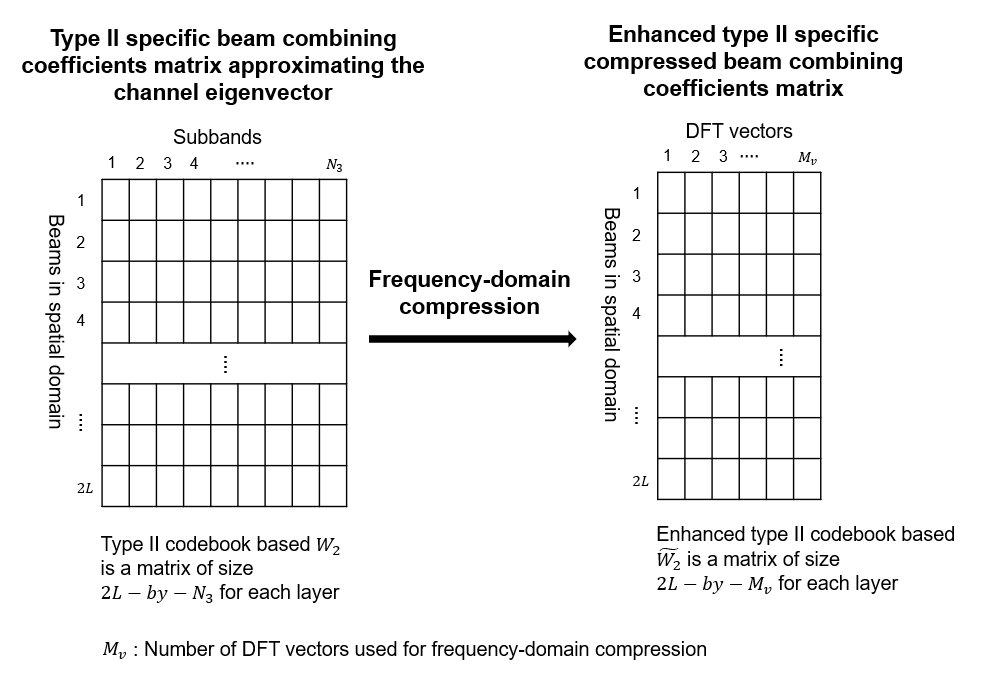Plot the subband SINR values and subband CQI values for each codeword for both practical and perfect channel estimation scenarios. The plot highlights the variations of SINR and CQI values across the subbands for a specified slot number (0-based), if CQI is reported in that slot. Otherwise, no plot is generated because the CQI is not reported.

The `plotSubbandCQIAndSINR` function plots the CQI values across all subbands only when the `CQIMode` is configured as `'Subband'`.

```% Provide slot number for which subband CQI values and PMI i2 indices must be plotted slotNum = 0; % Consider slot number as 0 (0-based) here, because CSI is % reported in that slot for the configured CSI-RS resource(s) plotSubbandCQIAndSINR(subbandCQIPractical,subbandCQIPerfect,SINRPerSubbandPerCWPractical,SINRPerSubbandPerCWPerfect,activeSlotNum,slotNum);```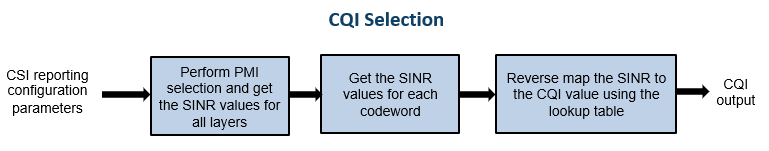#### Plot PMI and RI Indices

This section shows the plots that highlight the variations of PMI and RI indices due to channel fading conditions, for both practical channel estimation and perfect channel estimation scenarios. The plots show only the slots in which the CSI-RS is transmitted or in which PMI and RI are calculated.

When codebook type is type 1 single panel

The PMI indices are (${\mathit{i}}_{1}:\left[{\mathit{i}}_{1,1},{\mathit{i}}_{1,2},{\mathit{i}}_{1,3}\right]$ and ${\mathit{i}}_{2}$).

The first plot shows the rank and the corresponding PMI ${\mathit{i}}_{1}$ indices variation across multiple slots.

The second plot highlights the variation in ${\mathit{i}}_{2}$ indices across:

• The slots - When the `PMIMode` is configured as `'Wideband'`

• The subbands in the specified slot - When the `PMIMode` is configured as `'Subband'`

When codebook type is type 1 multipanel

The PMI indices are (${\mathit{i}}_{1}:\left[{\mathit{i}}_{1,1},{\mathit{i}}_{1,2},{\mathit{i}}_{1,3},{\mathit{i}}_{1,4,1},{\mathit{i}}_{1,4,2},{\mathit{i}}_{1,4,3}\right]$ and ${\mathit{i}}_{2}:\left[{\mathit{i}}_{2,0},{\mathit{i}}_{2,1},{\mathit{i}}_{2,2}\right]$).

In this case, the first two plots show the rank and the corresponding PMI ${\mathit{i}}_{1}$ indices (${\mathit{i}}_{1,1}$ to ${\mathit{i}}_{1,4,3}$) variation across multiple slots.

The third plot highlights the variation in each of the ${\mathit{i}}_{2}$ index (${\mathit{i}}_{2,0}$ to ${\mathit{i}}_{2,2}$) across:

• The slots - When the `PMIMode` is configured as `'Wideband'`

• The subbands in the specified slot - When the `PMIMode` is configured as `'Subband'`

```% Set the showPMIandRIPlots flag to true, if you want to see the plots for % the PMI and RI showPMIandRIPlots = true; if showPMIandRIPlots plotPMIAndRI(pmiPracticalPerSlot,pmiPerfectPerSlot,riPracticalPerSlot,riPerfectPerSlot,activeSlotNum,slotNum); end```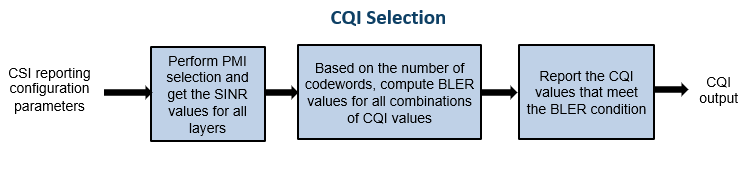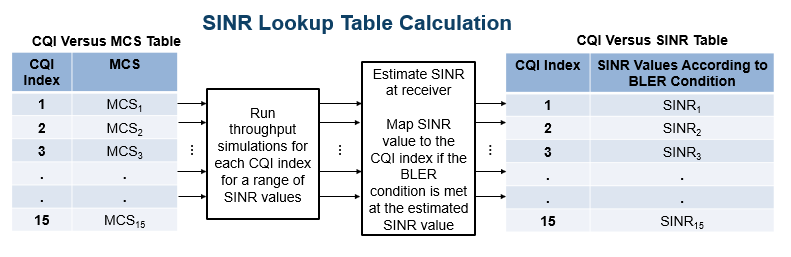### Summary and Further Exploration

The example shows how to compute downlink CSI parameters such as the CQI and PMI from type 1 single panel codebooks, and RI for a MIMO scenario with a TDL channel. The example also supports the computation of CSI parameters using type 1 multipanel codebooks.

You can modify carrier, channel, CSI-RS resource configurations, and CSI reporting configuration parameters (such as the codebook type, the mode of CQI and PMI reporting, and the subband size) and observe the variations in the computed CQI, PMI, and RI values across the time (slots) and frequency (subbands).

### References

1. 3GPP TS 38.214. "NR; Physical layer procedures for data." 3rd Generation Partnership Project; Technical Specification Group Radio Access Network.

### Local Functions

The example uses these local functions to validate the CSI-RS configuration object, and to plot the computed CQI, PMI, and RI values

```function validateCSIRSConfig(carrier,csirs,nTxAnts) % validateCSIRSConfig(CARRIER,CSIRS,NTXANTS) validates the CSI-RS % configuration, given the carrier specific configuration object CARRIER, % CSI-RS configuration object CSIRS, and the number of transmit antennas % NTXANTS. % Validate the number of CSI-RS ports if ~isscalar(unique(csirs.NumCSIRSPorts)) error('nr5g:InvalidCSIRSPorts',... 'All the CSI-RS resources must be configured to have the same number of CSI-RS ports.'); end % Validate the CDM lengths if ~iscell(csirs.CDMType) cdmType = {csirs.CDMType}; else cdmType = csirs.CDMType; end if (~all(strcmpi(cdmType,cdmType{1}))) error('nr5g:InvalidCSIRSCDMTypes',... 'All the CSI-RS resources must be configured to have the same CDM lengths.'); end if nTxAnts ~= csirs.NumCSIRSPorts(1) error('nr5g:InvalidNumTxAnts',['Number of transmit antennas (' num2str(nTxAnts)... ') must be equal to the number of CSI-RS ports (' num2str(csirs.NumCSIRSPorts(1)) ').']); end % Check for the overlap between the CSI-RS indices csirsInd = nrCSIRSIndices(carrier,csirs,"OutputResourceFormat",'cell'); numRes = numel(csirsInd); csirsIndAll = cell(1,numRes); ratioVal = csirs.NumCSIRSPorts(1)/prod(getCDMLengths(csirs)); for resIdx = 1:numRes if ~isempty(csirsInd{resIdx}) grid = nrResourceGrid(carrier,csirs.NumCSIRSPorts(1)); [~,tempInd] = nrExtractResources(csirsInd{resIdx},grid); if numel(tempInd)/numel(csirsInd{resIdx}) ~= ratioVal error('nr5g:OverlappedCSIRSREsSingleResource',['CSI-RS indices of resource '... num2str(resIdx) ' must be unique. Try changing the symbol or subcarrier locations.']); end csirsIndAll{resIdx} = tempInd(:); for idx = 1:resIdx-1 overlappedInd = ismember(csirsIndAll{idx},csirsIndAll{resIdx}); if any(overlappedInd) error('nr5g:OverlappedCSIRSREsMultipleResources',['The resource elements of the '... 'configured CSI-RS resources must not overlap. Try changing the symbol or '... 'subcarrier locations of CSI-RS resource ' num2str(idx) ' and resource ' num2str(resIdx) '.']); end end end end end function cdmLengths = getCDMLengths(csirs) % CDMLENGTHS = getCDMLengths(CSIRS) returns the CDM lengths given the % CSI-RS configuration object CSIRS. CDMType = csirs.CDMType; if ~iscell(csirs.CDMType) CDMType = {csirs.CDMType}; end CDMTypeOpts = {'noCDM','fd-CDM2','CDM4','CDM8'}; CDMLengthOpts = {[1 1],[2 1],[2 2],[2 4]}; cdmLengths = CDMLengthOpts{strcmpi(CDMTypeOpts,CDMType{1})}; end function [cqiPracticalPerSlot,subbandCQIPractical,pmiPracticalPerSlot,SINRPerSubbandPerCWPractical,cqiPerfectPerSlot,subbandCQIPerfect,pmiPerfectPerSlot,SINRPerSubbandPerCWPerfect,riPracticalPerSlot,riPerfectPerSlot] = fillInactiveSlots(cqiPracticalPerSlot,subbandCQIPractical,pmiPracticalPerSlot,SINRPerSubbandPerCWPractical,cqiPerfectPerSlot,subbandCQIPerfect,pmiPerfectPerSlot,SINRPerSubbandPerCWPerfect,riPracticalPerSlot,riPerfectPerSlot,reportConfig,totSlotsBinaryVec,activeSlots) % [CQIPRACTICALPERSLOT,SUBBANDCQIPRACTICAL,PMIPRACTICALPERSLOT,SINRPERSUBBANDPERCWPRACTICAL,CQIPERFECTPERSLOT,SUBBANDCQIPERFECT,PMIPERFECTPERSLOT,SINRPERSUBBANDPERCWPERFECT,RIPRACTICALPERSLOT,RIPERFECTPERSLOT] = fillInactiveSlots(CQIPRACTICALPERSLOT,SUBBANDCQIPRACTICAL,PMIPRACTICALPERSLOT,SINRPERSUBBANDPERCWPRACTICAL,CQIPERFECTPERSLOT,SUBBANDCQIPERFECT,PMIPERFECTPERSLOT,SINRPERSUBBANDPERCWPERFECT,RIPRACTICALPERSLOT,RIPERFECTPERSLOT,REPORTCONFIG,TOTSLOTSBINARYVEC,ACTIVESLOTS) % returns the CQI, PMI, and RI related variables filled with NaNs in the % slots where NZP-CSI-RS is not present according to the codebook type from % the report configuration structure REPORTCONFIG. % Note that the CQI, PMI and RI variables are returned as empty if there % are no NZP-CSI-RS resources, that is, no active slots in the entire % simulation duration. % Compute the indices of the slots and the number of slots in which % NZP-CSI-RS is not present inactiveSlotIdx = ~totSlotsBinaryVec; numInactiveSlots = nnz(inactiveSlotIdx); if ~isempty(activeSlots) numCQISBs = size(cqiPracticalPerSlot,1); % Get the codebook type codebookType = 'Type1SinglePanel'; if isfield(reportConfig,'CodebookType') codebookType = validatestring(reportConfig.CodebookType,{'Type1SinglePanel','Type1MultiPanel'},'fillInactiveSlots','CodebookType field'); end % Fill the CQI, PMI and RI variables with NaNs in the slots where NZP-CSI-RS is % not present cqiPracticalPerSlot(:,:,inactiveSlotIdx) = NaN(numCQISBs,2,numInactiveSlots); subbandCQIPractical(:,:,inactiveSlotIdx) = NaN(numCQISBs,2,numInactiveSlots); SINRPerSubbandPerCWPractical(:,:,inactiveSlotIdx) = NaN(numCQISBs,2,numInactiveSlots); cqiPerfectPerSlot(:,:,inactiveSlotIdx) = NaN(numCQISBs,2,numInactiveSlots); subbandCQIPerfect(:,:,inactiveSlotIdx) = NaN(numCQISBs,2,numInactiveSlots); SINRPerSubbandPerCWPerfect(:,:,inactiveSlotIdx) = NaN(numCQISBs,2,numInactiveSlots); riPracticalPerSlot(inactiveSlotIdx) = NaN; riPerfectPerSlot(inactiveSlotIdx) = NaN; numi1Indices = 3; numi2Indices = 1; if strcmpi(codebookType,'Type1MultiPanel') numi1Indices = 6; numi2Indices = 3; end numPMISBs = size(pmiPerfectPerSlot(activeSlots(1)).i2,2); [pmiPerfectPerSlot(inactiveSlotIdx),pmiPracticalPerSlot(inactiveSlotIdx)] = deal(struct('i1',NaN(1,numi1Indices),'i2',NaN(numi2Indices,numPMISBs))); end end function plotWidebandCQIAndSINR(cqiPracticalPerSlot,cqiPerfectPerSlot,SINRPerSubbandPerCWPractical,SINRPerSubbandPerCWPerfect,activeSlotNum) % plotWidebandCQIAndSINR(CQIPRACTICALPERSLOT,CQIPERFECTPERSLOT,SINRPERSUBBANDPERCWPRACTICAL,SINRPERSUBBANDPERCWPERFECT,ACTIVESLOTNUM) % plots the wideband SINR and wideband CQI values for each codeword % across all specified active slots ACTIVESLOTNUM (1-based) (in which the % CQI is reported as other than NaN) for practical and perfect channel % estimation cases. % Check if there are no slots in which NZP-CSI-RS is present if isempty(activeSlotNum) disp('No CQI data to plot, because there are no slots in which NZP-CSI-RS is present.'); return; end if all(isnan(cqiPracticalPerSlot(1,2,activeSlotNum))) numCW = 1; else numCW = 2; end cqiPracticalPerCW = permute(cqiPracticalPerSlot(1,:,:),[1 3 2]); cqiPerfectPerCW = permute(cqiPerfectPerSlot(1,:,:),[1 3 2]); SINRPerCWPractical = permute(SINRPerSubbandPerCWPractical(1,:,:),[1 3 2]); SINRPerCWPerfect = permute(SINRPerSubbandPerCWPerfect(1,:,:),[1 3 2]); % Extract wideband CQI indices for slots where NZP-CSI-RS is present cqiPracticalPerCWActiveSlots = cqiPracticalPerCW(1,activeSlotNum,:); cqiPerfectPerCWActiveSlots = cqiPerfectPerCW(1,activeSlotNum,:); widebandSINRPractical = 10*log10(SINRPerCWPractical(1,activeSlotNum,:)); widebandSINRPerfect = 10*log10(SINRPerCWPerfect(1,activeSlotNum,:)); xTickLables = num2cell(activeSlotNum(:)-1); if isempty(reshape(cqiPracticalPerCWActiveSlots(:,:,1),1,[])) disp('No CQI data to plot, because all CQI values are NaNs.'); return; end figure(); subplot(211) plot(widebandSINRPerfect(:,:,1),'ro-') hold on; plot(widebandSINRPractical(:,:,1),'b*-') if numCW == 2 hold on; plot(widebandSINRPerfect(:,:,2),'rs:') hold on; plot(widebandSINRPractical(:,:,2),'bd:') title('Estimated Wideband SINR Values for Codeword 1&2'); xlabel('Slots'); ylabel('Wideband SINR Values in dB'); xticks(1:size(cqiPracticalPerCWActiveSlots,2)); xticklabels(xTickLables); [lowerBound,upperBound] = bounds([widebandSINRPractical(:);widebandSINRPerfect(:)],'omitnan'); ylim([lowerBound-1 upperBound+3.5]); legend({'Codeword 1:Perfect channel est.','Codeword 1:Practical channel est.','Codeword 2:Perfect channel est.','Codeword 2:Practical channel est.'}); else title('Estimated Wideband SINR Values for Codeword 1'); xlabel('Slots'); ylabel('Wideband SINR Values in dB'); xticks(1:size(cqiPracticalPerCWActiveSlots,2)); xticklabels(xTickLables); [lowerBound,upperBound] = bounds([widebandSINRPractical(:);widebandSINRPerfect(:)]); ylim([lowerBound-1 upperBound+3.5]); legend({'Codeword 1:Perfect channel est.','Codeword 1:Practical channel est.'}); end subplot(212) plot(cqiPerfectPerCWActiveSlots(:,:,1),'r-o'); hold on; plot(cqiPracticalPerCWActiveSlots(:,:,1),'b-*'); if numCW == 2 hold on; plot(cqiPerfectPerCWActiveSlots(:,:,2),'r:s'); hold on; plot(cqiPracticalPerCWActiveSlots(:,:,2),'b:d'); xlabel('Slots'); xticks(1:size(cqiPracticalPerCWActiveSlots,2)); xticklabels(xTickLables); ylabel('Wideband CQI'); [lowerBound,upperBound] = bounds([cqiPracticalPerCWActiveSlots(:);cqiPerfectPerCWActiveSlots(:)]); ylim([lowerBound-1 upperBound+3.5]); title('Wideband CQI Values for Codeword 1&2'); legend({'Codeword 1:Perfect channel est.','Codeword 1:Practical channel est.','Codeword 2:Perfect channel est.','Codeword 2:Practical channel est.'}); else xlabel('Slots'); xticks(1:size(cqiPracticalPerCWActiveSlots,2)); xticklabels(xTickLables); ylabel('Wideband CQI'); [lowerBound,upperBound] = bounds([cqiPracticalPerCWActiveSlots(:,:,1)';cqiPerfectPerCWActiveSlots(:,:,1)']); ylim([lowerBound-1 upperBound+3.5]); title('Wideband CQI Values for Codeword 1'); legend({'Codeword 1:Perfect channel est.','Codeword 1:Practical channel est.'}); end end function plotSubbandCQIAndSINR(subbandCQIPractical,subbandCQIPerfect,SINRPerCWPractical,SINRPerCWPerfect,activeSlotNum,nslot) % plotSUBBANDCQIAndSINR(SUBBANDCQIPRACTICAL,SUBBANDCQIPERFECT,SINRPERCWPRACTICAL,SINRPERCWPERFECT,ACTIVESLOTNUM,NSLOT) % plots the SINR and CQI values for each codeword across all of the % subbands for practical and perfect channel estimation cases for the % given slot NSLOT (0-based) among all specified active slots % ACTIVESLOTNUM (1-based). The function does not plot the values if % CQIMode is 'Wideband' or if the CQI and SINR values are all NaNs in the % given NSLOT. % Check if there are no slots in which NZP-CSI-RS is present if isempty(activeSlotNum) disp('No CQI data to plot, because there are no slots in which NZP-CSI-RS is present.'); return; end if all(isnan(subbandCQIPractical(:,2,activeSlotNum))) numCW = 1; else numCW = 2; end numSubbands = size(subbandCQIPractical,1); if numSubbands > 1 Mode = 'Subband'; else Mode = 'Wideband'; end % Plot subband CQI values if strcmpi(Mode,'subband') subbandCQIPerCWPractical = subbandCQIPractical(2:end,:,nslot+1); subbandCQIPerCWPerfect = subbandCQIPerfect(2:end,:,nslot+1); subbandSINRPerCWPractical = 10*log10(SINRPerCWPractical(2:end,:,nslot+1)); subbandSINRPerCWPerfect = 10*log10(SINRPerCWPerfect(2:end,:,nslot+1)); xTickLables = num2cell(1:size(subbandCQIPerfect,1)-1); % Excluding the value of wideband if ~any(nslot+1 == activeSlotNum) disp(['For the specified slot (' num2str(nslot) '), CQI values are not reported. Please choose another slot number.']) else figure(); subplot(211) plot(subbandSINRPerCWPerfect(:,1),'ro-') hold on; plot(subbandSINRPerCWPractical(:,1),'b*-') if numCW == 2 hold on; plot(subbandSINRPerCWPerfect(:,2),'rs:') hold on; plot(subbandSINRPerCWPractical(:,2),'bd:') title(['Estimated Subband SINR Values for Codeword 1&2 in Slot ' num2str(nslot)]); xlabel('Subbands'); ylabel('Subband SINR Values in dB'); xticks(1:numSubbands); xticklabels(xTickLables); xlim([0 numSubbands+1]); [lowerBound,upperBound] = bounds([subbandSINRPerCWPerfect(:);subbandSINRPerCWPractical(:)],'omitnan'); ylim([lowerBound-1 upperBound+3.5]); legend({'Codeword 1:Perfect channel est.','Codeword 1:Practical channel est.','Codeword 2:Perfect channel est.','Codeword 2:Practical channel est.'}); else title(['Estimated Subband SINR Values for Codeword 1 in Slot ' num2str(nslot)]); xlabel('Subbands'); ylabel('Subband SINR Values in dB'); xticks(1:numSubbands); xticklabels(xTickLables); xlim([0 numSubbands+1]); [lowerBound,upperBound] = bounds([subbandSINRPerCWPerfect(:,1);subbandSINRPerCWPractical(:,1)]); ylim([lowerBound-1 upperBound+3.5]); legend({'Codeword 1:Perfect channel est.','Codeword 1:Practical channel est.'}); end subplot(212) plot(subbandCQIPerCWPerfect(:,1),'r-o'); hold on; plot(subbandCQIPerCWPractical(:,1),'b-*'); if numCW == 2 hold on; plot(subbandCQIPerCWPerfect(:,2),'r:s'); hold on; plot(subbandCQIPerCWPractical(:,2),'b:d'); title(['Subband CQI Values for Codeword 1&2 in Slot ' num2str(nslot)]); xlabel('Subbands'); ylabel('Subbands CQI'); xticks(1:numSubbands); xticklabels(xTickLables); xlim([0 numSubbands+1]); [lowerBound,upperBound] = bounds([subbandCQIPerCWPerfect(:);subbandCQIPerCWPractical(:)],'omitnan'); ylim([lowerBound-1 upperBound+3.5]); legend({'Codeword 1:Perfect channel est.','Codeword 1:Practical channel est.','Codeword 2:Perfect channel est.','Codeword 2:Practical channel est.'}); else title(['Subband CQI Values for Codeword 1 in Slot ' num2str(nslot)]); xlabel('Subbands'); ylabel('Subbands CQI'); xticks(1:numSubbands); xticklabels(xTickLables); xlim([0 numSubbands+1]); [lowerBound,upperBound] = bounds([subbandCQIPerCWPerfect(:,1);subbandCQIPerCWPractical(:,1)]); ylim([lowerBound-1 upperBound+3.5]); legend({'Codeword 1:Perfect channel est.','Codeword 1:Practical channel est.'}); end end end end function plotPMIAndRI(pmiPracticalPerSlot,pmiPerfectPerSlot,riPracticalPerSlot,riPerfectPerSlot,activeSlotNum,nslot) % plotPMIAndRI(PMIPRACTICALPERSLOT,PMIPERFECTPERSLOT,RIPRACTICALPERSLOT,RIPERFECTPERSLOT,ACTIVESLOTNUM,NSLOT) % plots the RI and PMI i1 indices across all specified active slots % ACTIVESLOTNUM (1-based), for practical and perfect channel estimation % scenarios. The function also plots the i2 indices of practical and % perfect channel estimation scenarios across all specified active slots % when the PMI mode is 'Wideband' or plots i2 indices across all of the % subbands for the specified slot number NSLOT (0-based) when the PMI % mode is 'Subband'. % Check if there are no slots in which NZP-CSI-RS is present if isempty(activeSlotNum) disp('No PMI and RI data to plot, because there are no slots in which NZP-CSI-RS is present.'); return; end % Get number of active slots numActiveSlots = numel(activeSlotNum); numi1Indices = numel(pmiPracticalPerSlot(activeSlotNum(1)).i1); if numi1Indices == 6 codebookType = 'Type1MultiPanel'; else codebookType = 'Type1SinglePanel'; end % Extract wideband PMI indices (i1 values) for slots where NZP-CSI-RS % is present i1PerfectValsActiveSlots = reshape([pmiPerfectPerSlot(activeSlotNum).i1],numi1Indices,[])'; i1PracticalValsActiveSlots = reshape([pmiPracticalPerSlot(activeSlotNum).i1],numi1Indices,[])'; % Extract RI values for slots where NZP-CSI-RS is present RIPerfectValsActiveSlots = riPerfectPerSlot(activeSlotNum)'; RIPracticalValsActiveSlots = riPracticalPerSlot(activeSlotNum)'; if isempty(i1PerfectValsActiveSlots) disp('No PMI and RI data to plot, because all PMI and RI values are NaNs.'); return; end figure; subplot(4,1,1) plot(RIPerfectValsActiveSlots,'r-o'); hold on; plot(RIPracticalValsActiveSlots,'b-*'); xlabel('Slots') ylabel('RI Values'); xticks(1:numActiveSlots); xTickLables = num2cell(activeSlotNum(:)-1); xticklabels(xTickLables); [~,upperBound] = bounds([RIPerfectValsActiveSlots; RIPracticalValsActiveSlots]); xlim([0 numActiveSlots+8]); ylim([0 upperBound+2]); yticks(0:upperBound+2); title('RI Values') legend({'Perfect channel est.','Practical channel est.'}); % Get i11 indices i11PerfectVals = i1PerfectValsActiveSlots(:,1); i11PracticalVals = i1PracticalValsActiveSlots(:,1); % Plot i11 values subplot(4,1,2) plot(i11PerfectVals,'r-o'); hold on; plot(i11PracticalVals,'b-*'); xlabel('Slots') ylabel('i11 Indices'); xticks(1:numActiveSlots); xticklabels(xTickLables); [lowerBound,upperBound] = bounds([i11PerfectVals; i11PracticalVals]); xlim([0 numActiveSlots+8]); ylim([lowerBound-2 upperBound+2]); title('PMI: i11 Indices') legend({'Perfect channel est.','Practical channel est.'}); % Get i12 indices i12PerfectVals = i1PerfectValsActiveSlots(:,2); i12PracticalVals = i1PracticalValsActiveSlots(:,2); % Plot i12 values subplot(4,1,3) plot(i12PerfectVals,'r-o'); hold on; plot(i12PracticalVals,'b-*'); xlabel('Slots') ylabel('i12 Indices'); xticks(1:numActiveSlots); xticklabels(xTickLables); [lowerBound,upperBound] = bounds([i12PerfectVals; i12PracticalVals]); xlim([0 numActiveSlots+8]); ylim([lowerBound-2 upperBound+2]); title('PMI: i12 Indices') legend({'Perfect channel est.','Practical channel est.'}); % Get i13 indices i13PerfectVals = i1PerfectValsActiveSlots(:,3); i13PracticalVals = i1PracticalValsActiveSlots(:,3); % Plot i13 values subplot(4,1,4) plot(i13PerfectVals,'r-o'); hold on; plot(i13PracticalVals,'b-*'); xlabel('Slots') ylabel('i13 Indices'); xticks(1:numActiveSlots); xticklabels(xTickLables); [lowerBound,upperBound] = bounds([i13PerfectVals; i13PracticalVals]); xlim([0 numActiveSlots+8]); ylim([lowerBound-2 upperBound+2]); title('PMI: i13 Indices') legend({'Perfect channel est.','Practical channel est.'}); % Plot the i141, i142 and i143 indices in type 1 multipanel case if strcmpi(codebookType,'Type1MultiPanel') figure() % Get i141 indices i141PerfectVals = i1PerfectValsActiveSlots(:,4); i141PracticalVals = i1PracticalValsActiveSlots(:,4); % Plot i141 values subplot(3,1,1) plot(i141PerfectVals,'r-o'); hold on; plot(i141PracticalVals,'b-*'); xlabel('Slots') ylabel('i141 Indices'); xticks(1:numActiveSlots); xticklabels(xTickLables); [lowerBound,upperBound] = bounds([i141PerfectVals; i141PracticalVals]); xlim([0 numActiveSlots+8]); ylim([lowerBound-2 upperBound+2]); title('PMI: i141 Indices') legend({'Perfect channel est.','Practical channel est.'}); % Get i142 indices i142PerfectVals = i1PerfectValsActiveSlots(:,5); i142PracticalVals = i1PracticalValsActiveSlots(:,5); % Plot i142 values subplot(3,1,2) plot(i142PerfectVals,'r-o'); hold on; plot(i142PracticalVals,'b-*'); xlabel('Slots') ylabel('i142 Indices'); xticks(1:numActiveSlots); xticklabels(xTickLables); [lowerBound,upperBound] = bounds([i142PerfectVals; i142PracticalVals]); xlim([0 numActiveSlots+8]); ylim([lowerBound-2 upperBound+2]); title('PMI: i142 Indices') legend({'Perfect channel est.','Practical channel est.'}); % Get i143 indices i143PerfectVals = i1PerfectValsActiveSlots(:,6); i143PracticalVals = i1PracticalValsActiveSlots(:,6); % Plot i143 values subplot(3,1,3) plot(i143PerfectVals,'r-o'); hold on; plot(i143PracticalVals,'b-*'); xlabel('Slots') ylabel('i143 Indices'); xticks(1:numActiveSlots); xticklabels(xTickLables); [lowerBound,upperBound] = bounds([i143PerfectVals; i143PracticalVals]); xlim([0 numActiveSlots+8]); ylim([lowerBound-2 upperBound+2]); title('PMI: i143 Indices') legend({'Perfect channel est.','Practical channel est.'}); end numSubbands = size(pmiPracticalPerSlot(activeSlotNum(1)).i2,2); % Get the number of i2 indices according to codebook type numi2Indices = 1; if strcmpi(codebookType,'Type1MultiPanel') numi2Indices = 3; end % Extract i2 values i2PerfectVals = reshape([pmiPerfectPerSlot(activeSlotNum).i2],[numSubbands,numi2Indices,numActiveSlots]); % Of size numActiveSlots-by-numi2Indices-numSubbands i2PracticalVals = reshape([pmiPracticalPerSlot(activeSlotNum).i2],[numSubbands,numi2Indices,numActiveSlots]); % Of size numActiveSlots-by-numi2Indices-numSubbands if numSubbands > 1 mode = 'Subband'; else mode = 'Wideband'; end % Plot i2 values if strcmpi(mode,'Wideband') figure; % In type 1 single panel case, there is only one i2 index. The % first column of i2PerfectVals and i2PracticalVals corresponds to % i2 index. In type 1 multipanel case, there i2 values are a set % of three indices i20, i21 and i22. The first column of in this % case i2PerfectVals and i2PracticalVals corresponds to i20 index. % Extract the respective index values i20PerfectVals = reshape(i2PerfectVals(:,1,:),[],numActiveSlots).'; i20PracticalVals = reshape(i2PracticalVals(:,1,:),[],numActiveSlots).'; % Plot i2 values or i20 values respectively subplot(numi2Indices,1,1) plot(i20PerfectVals,'r-o'); hold on; plot(i20PracticalVals,'b-*'); if strcmpi(codebookType,'Type1SinglePanel') title('PMI: i2 Indices') xlabel('Slots') ylabel('i2 Indices'); else title('PMI: i20 Indices') xlabel('Slots') ylabel('i20 Indices'); end xticks(1:numActiveSlots); xticklabels(xTickLables); [lowerBound,upperBound] = bounds([i20PerfectVals; i20PracticalVals]); xlim([0 numActiveSlots+8]); ylim([lowerBound-1 upperBound+1]); legend({'Perfect channel est.','Practical channel est.'}); if strcmpi(codebookType,'Type1MultiPanel') % Get i21 values in each slot i21PerfectVals = reshape(i2PerfectVals(:,2,:),[],numActiveSlots).'; i21PracticalVals = reshape(i2PracticalVals(:,2,:),[],numActiveSlots).'; % Plot i21 values subplot(numi2Indices,1,2) plot(i21PerfectVals,'r-o'); hold on; plot(i21PracticalVals,'b-*'); title('PMI: i21 Indices') xlabel('Slots') ylabel('i21 Indices'); xticks(1:numActiveSlots); xticklabels(xTickLables); [lowerBound,upperBound] = bounds([i21PerfectVals; i21PracticalVals]); xlim([0 numActiveSlots+8]); ylim([lowerBound-1 upperBound+1]); legend({'Perfect channel est.','Practical channel est.'}); % Get i22 values in each slot i22PerfectVals = reshape(i2PerfectVals(:,3,:),[],numActiveSlots).'; i22PracticalVals = reshape(i2PracticalVals(:,3,:),[],numActiveSlots).'; % Plot i22 values subplot(numi2Indices,1,3) plot(i22PerfectVals,'r-o'); hold on; plot(i22PracticalVals,'b-*'); title('PMI: i22 Indices') xlabel('Slots') ylabel('i22 Indices'); xticks(1:numActiveSlots); xticklabels(xTickLables); [lowerBound,upperBound] = bounds([i22PerfectVals; i22PracticalVals]); xlim([0 numActiveSlots+8]); ylim([lowerBound-1 upperBound+1]); legend({'Perfect channel est.','Practical channel est.'}); end else if any(nslot+1 == activeSlotNum) % In subband mode, plot the PMI i2 indices corresponding to the % specified slot number figure; % Plot i20 values subplot(numi2Indices,1,1) plot(pmiPerfectPerSlot(nslot+1).i2(1,:),'r-o'); hold on; plot(pmiPracticalPerSlot(nslot+1).i2(1,:),'b-*'); if strcmpi(codebookType,'Type1SinglePanel') title(['PMI: i2 Indices for All Subbands in Slot ' num2str(nslot)]); xlabel('Subbands') ylabel('i2 Indices'); else title(['PMI: i20 Indices for All Subbands in Slot ' num2str(nslot)]); xlabel('Subbands') ylabel('i20 Indices'); end xticks(1:numSubbands); xticklabels(num2cell(1:numSubbands)); [lowerBound,upperBound] = bounds([pmiPerfectPerSlot(nslot+1).i2(1,:) pmiPracticalPerSlot(nslot+1).i2(1,:)]); yticks(lowerBound:upperBound); yticklabels(num2cell(lowerBound:upperBound)); xlim([0 numSubbands+1]) ylim([lowerBound-1 upperBound+1]); legend({'Perfect channel est.','Practical channel est.'}); if strcmpi(codebookType,'Type1MultiPanel') % Plot i21 values subplot(numi2Indices,1,2) plot(pmiPerfectPerSlot(nslot+1).i2(2,:),'r-o'); hold on; plot(pmiPracticalPerSlot(nslot+1).i2(2,:),'b-*'); title(['PMI: i21 Indices for All Subbands in Slot ' num2str(nslot)]); xlabel('Subbands') ylabel('i21 Indices'); xticks(1:numSubbands); xticklabels(num2cell(1:numSubbands)); [lowerBound,upperBound] = bounds([pmiPerfectPerSlot(nslot+1).i2(2,:) pmiPracticalPerSlot(nslot+1).i2(2,:)]); yticks(lowerBound:upperBound); yticklabels(num2cell(lowerBound:upperBound)); xlim([0 numSubbands+1]) ylim([lowerBound-1 upperBound+1]); legend({'Perfect channel est.','Practical channel est.'}); % Plot i22 values subplot(numi2Indices,1,3) plot(pmiPerfectPerSlot(nslot+1).i2(3,:),'r-o'); hold on; plot(pmiPracticalPerSlot(nslot+1).i2(3,:),'b-*'); title(['PMI: i22 Indices for All Subbands in Slot ' num2str(nslot)]); xlabel('Subbands') ylabel('i22 Indices'); xticks(1:numSubbands); xticklabels(num2cell(1:numSubbands)); [lowerBound,upperBound] = bounds([pmiPerfectPerSlot(nslot+1).i2(3,:) pmiPracticalPerSlot(nslot+1).i2(3,:)]); yticks(lowerBound:upperBound); yticklabels(num2cell(lowerBound:upperBound)); xlim([0 numSubbands+1]) ylim([lowerBound-1 upperBound+1]); legend({'Perfect channel est.','Practical channel est.'}); end else disp(['For the specified slot (' num2str(nslot) '), PMI i2 indices are not reported. Please choose another slot number.']) end end end```